# VBA Arrays

An array is a structure that can store a multidimensional list of data. A variable can store a single element.

An array can store multiple elements referenced by index numbers

## 0. Preliminary

• A one dimension array is like a list (vector) of data, while a two dimensional array is like a table of data or a matrix
• VBA arrays can have up to 60 dimensions. Generally, three dimension is the maximum required in the business environment. The dimension can be conceptualized as 1. rows, 2. columns, and 3. depth (equivalent to multi worksheets in Excel)
• The number of elements in each dimension is only limited by computer memory. For example, you could have a 10,000 by 100,000 array
• Arrays are very useful for data processing as each individual item can be accessed via its index number(s)
• Details of the elements of an array can be viewed in the Locals Window (in Step or Break mode) or other techniques such as Debug.Print

## 1. An array can either be static or dynamic

An array is declared in a similar way to a variable, and follows the same naming conventions. Note: you cannot use the name Array, because Array in a VBA keyword (a function).

### 1.1 Static array

A static array is given a fixed number of elements at the point of declaration. In other words the analyst needs to know the array dimension before the code is written.

Code 1a: DemoStatic1 commas separate the dimensions. The values are the upper index value of the dimension.

Base 0 by default
Sub DemoStatic1()

' Static array - includes the dimension n, in the brackets (n)
Dim Price1D(5) As Double      ' one dimension with elements 0 to 5
Dim Price2D(3, 1) As Variant  ' two dimensions with elements 0 to 3, and 0 to 1

' Assign some values
Price1D(0) = 10
Price1D(4) = 50

' Populate selected elements
Price2D(0, 0) = "Coy1"
Price2D(0, 1) = 123.45
Price2D(3, 0) = "Coy4"
Price2D(3, 1) = 456.78

End Sub


A static array includes the dimension, and the upper index number elements in the dimension in declaration (Dim) statement. A one dimensional array (row vector), example Price1D in code 1 has no commas in the brackets; a two dimensional array, example Price2D in code 1 has one comma separating the dimension, a three dimensional arrays has two commas, .... A two dimensional array is similar to a worksheet viewed in R1C1 reference style. Cell/element coordinates are denoted by the row, column pair (r, c).

When viewed in Break mode, the code 1 arrays are shown in the Locals Window (figure 1).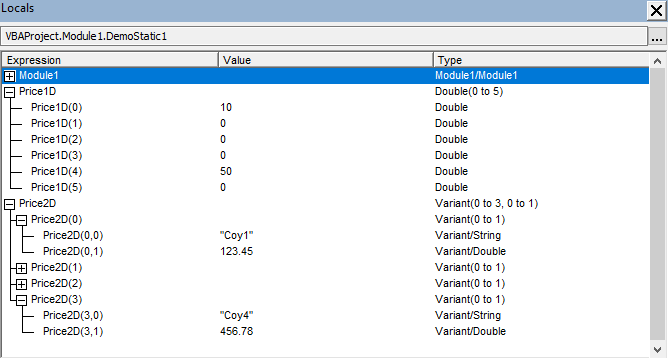Fig 1: Locals Window static array declaration and population of elements

Price1D has only one dimension and is equivalent to a row vector. The first element, index 0 has value 10. The fifth element, index 4 has value 50.

### Option Base statement

By default, the lower index number of the array dimension is zero.

• If you define an array with upper index 5 (see code 1, line 8), the indices will be 0,1,2,3,4,5 not 1,2,3,4,5
• You can change the starting point from zero to one (set the lower index to 1) by including the following statement in the Declaration area at the top of the module

• Option Base 1 (see code 1b line 1), or
• Explicitly set the lower bound in the declaration with the To statement (see code 1c, lines 8 and 9)

• Option Base is module specific
• Array dimension index numbers can be any consecutive sequence on the integer number line
• Generally, the base is usually 0 or 1, but could be negative (see Dim shpArray(-2 to 24)), or even match a value like the year Dim TaxR(2010 To 2016, 1 To 5, 1 To 3)

Code 1b: DemoStatic1 commas separate the dimensions. The values in the brackets are the upper index value of the dimension.

Explicit Option Base 1 declaration
Option Base 1

Sub DemoStatic1()

' Static array - includes the dimension n, in the brackets (n)
Dim Price1D(5) As Double      ' one dimension with elements 1 to 5
Dim Price2D(3, 1) As Variant  ' two dimensions with elements 1 to 3, and 1 to 1
' Price2D is now equivalent to a column vector with 3 rows and 1 column

' The assignment statement must reference valid index numbers
' < more code >
'


Code 1c: DemoStatic1 commas separate the dimensions. The values are the upper index value of the dimension.

Explicit lower bound declaration (Dim) with the To keyword
Sub DemoStatic1()

' Static array - includes the dimension n, in the brackets (n)
Dim Price1D(1 To 5) As Double      ' one dimension with elements 1 to 5
Dim Price2D(1 To 3, 1 To 1) As Variant  ' two dimensions with elements 1 to 3, and 1 to 1
' Price2D is now equivalent to a column vector with 3 rows and 1 column

' The assignment statement must reference valid index numbers
' < more code >
'


Other example of static arrays are in the next code module.

Static arrays :: sample code
Option Base 1

Sub DemoStaticArr1()
' Static array :: one dimension with upper index number
Dim first_array(5) As Integer   ' declare an array index 1 to 5
Dim a As Integer, x As Integer

a = 4
For x = 1 To 5
first_array(x) = x ' assign the index number as the value of each element
Next x

MsgBox "The value of first_array(" & a & ") is " & first_array(a)  ' return the value from the ath element
End Sub

' The array and the output is shown in figure 2

Sub DemoStaticArr2()
'   Static array :: multidimensional with lower and upper bound
Dim second_array(1 To 12, 1 To 10) As Integer  ' 2D array :: equivalent to 12 rows and 10 columns
Dim a As Integer, b As Integer
Dim x As Integer, y As Integer  ' loop counters

a = 5: b = 7
For x = 1 To 12
For y = 1 To 10
second_array(x, y) = x * y ' assign the product of the row and column numbers as the value of each element
Next y
Next x

MsgBox "The value of second_array(" & a & "," & b & ") is " & second_array(a, b)  ' return the value from the a,b element
End Sub

' The array and the output is shown in figure 3


Sub DemoStaticArr3()
' Static array :: one dimension with lower and upper bound
' Assign an array of strings

Dim third_array(0 To 5) As String   ' the lower band zero overrides the option base 1 declaration
Dim x As Integer: x = 3

third_array(0) = "Collingwood"
third_array(1) = "Essendon"
third_array(2) = "Fremantle"
third_array(3) = "Geelong"
third_array(4) = "Sydney Swans"

MsgBox "The value of third_array(" & x & ") is " & third_array(x)  ' return the value from the xth element)

End Sub

' The array and the output is shown in figure 4


### 1.2 Dynamic array

It often happens that the required size of the array is unknown at the time that the code is written. The solution is to declare a dynamic array whose dimension can be varied within the procedure. A dynamic array normally involves a two stage process.

1. Declare the array without dimension to reserve the name - use the Dim statement and an array with empty brackets (code 2a line 108)
2. Redimension the array to the required size using the Redim statement

A dynamic array can have its dimension changed whilst the code is running (within certain limits) - see code 2a.

### Redim statement

According to the statement syntax, the ReDim statement is used to size or resize a dynamic array already declared with a Dim statement

• SYNTAX: ReDim [Preserve] arrayname(subscripts)

where Preserve is optional, and subscripts represents the dimension of the array. See code 2a line 114

Code 2a: DemoDynamic1 Step 1: The Dim statement reserves the name. Step 2: The Redim statement creates the elements allowing data assignment
Sub DemoDynamic1()

' Dynamic array - no dimension at the point of declaration. The brackets are empty ()
Dim Price() As Double      ' this statement reserves the name Price in computer memory

' Suppose that the analyst determines that the array needs to have 7 elements
' This is performed with the ReDim statement

Redim Price(1 to 7)     ' the reserved name Price is assigned a dimension of 7 elements. Equivalent to a row vector
' the array data type cannot be changed with ReDim so Price is of the type Double

' Populate selected elements
Price(1) = 10#
Price(3) = 33.33
Price(5) = 55#
Price(7) = 70.7

End Sub


An alternate undocumented technique for the creation and dimension of a dynamic array is described by Getz and Gilbert (2006, p.379). This involves use of the ReDim statement to declare (create) the array. See code 2b.

### Preserve keyword

The ReDim statement erases all existing data in the array. All elements are reset to their default values, such as 0 for numeric type like Integer, and Double, and "" for String type array elements. To retain (preserve) the existing values when the array is resized, include the Preserve keyword. See code 2b line 146.

Code 2b: DemoDynamic2 ReDim one stage - combined declaration and size operation
Sub DemoDynamic2()

ReDim Price(1 to 7) As Double    ' declare and dimension a dynamic array in the procedure declaration
' the ReDim declaration / dimension statement is still valid within an Option Explicit declaration module
' Populate selected elements
Price(1) = 10#
Price(3) = 33.33
Price(5) = 55#
Price(7) = 70.7

1:      Stop    ' see figure 5        ' numeric colon is a label and is only used for reference purposes in the associated figures
Redim Preserve Price(1 to 9)
Price(9) = 90.9
2:      Stop    ' see figure 6

End Sub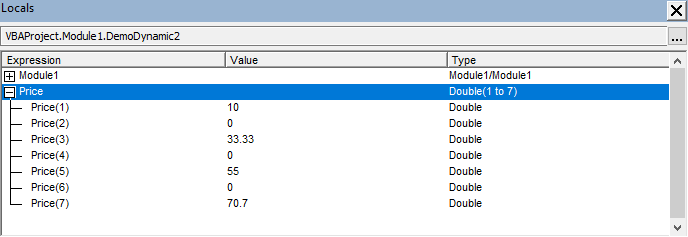Fig 5: Locals Window Price array - 7 elements at code line 145 Stop 1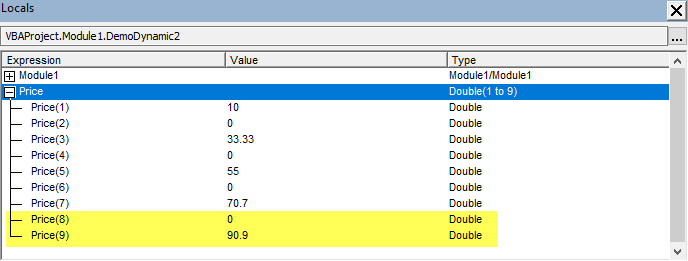Fig 6: Locals Window Price array - 9 elements at code line 148 Stop 2. The Redim statement increases the number of elements from 7 to 9. New elements 8 and 9 shown in yellow. The preserve statement retains the existing data

### LBound and UBound functions

Description and Syntax:

VBA function Description / argument
LBound(arrayname [,dimension])Returns a long for the smallest index value of the array dimension
dimension (optional): the array dimension - default 1 if omitted
UBound(arrayname [,dimension])Returns a long for the largest index value of the array dimension
dimension (optional): the array dimension - default 1 if omitted

The LBound and UBound functions are demonstrated in DemoDynamic7 and figure 9 below.

Dynamic arrays :: sample code
Option Base 1

Sub DemoDynamicArr4()
' Dynamic array :: 2 dimension with upper index numbers as variables
Dim fourth_array() As Integer     ' declare a dynamic array
Dim x As Integer, y As Integer
Dim a As Integer, b As Integer: a = 4: b = 3

ReDim fourth_array(a, b) As Integer ' Size :: 1st dimension 1 to a, 2nd dimension 1 to b (Option base 1)

For x = 1 To a
For y = 1 To b
fourth_array(x, y) = x * y
Next y
Next x

MsgBox "The value of fourth_array(" & a & "," & b & ") is " & fourth_array(a, b)  ' return the value from the a,b element
End Sub




Sub DemoDynamicArr5()
' Dynamic array :: 1. Declare a dynamic array
'                  2. Apply a size with the ReDim statement
'                  3. Populate the array
'                  4. Resize both dimension of the populated array
'                  5. Return a value

Dim fifth_array() As Integer    ' declare a dynamic array
Dim x As Integer, y As Integer
Dim a As Integer, b As Integer: a = 4: b = 3

ReDim fifth_array(a, b) As Integer

For x = 1 To a
For y = 1 To b
fifth_array(x, y) = x * y
Next y
Next x

1:  Stop
ReDim fifth_array(a + 2, b + 2) As Integer

2: Stop
MsgBox "The value of fifth_array(" & a & "," & b & ") is " & fifth_array(a, b)  ' return the value from the a,b element
End Sub

' The array at two Break Points, and the output is shown in figure 7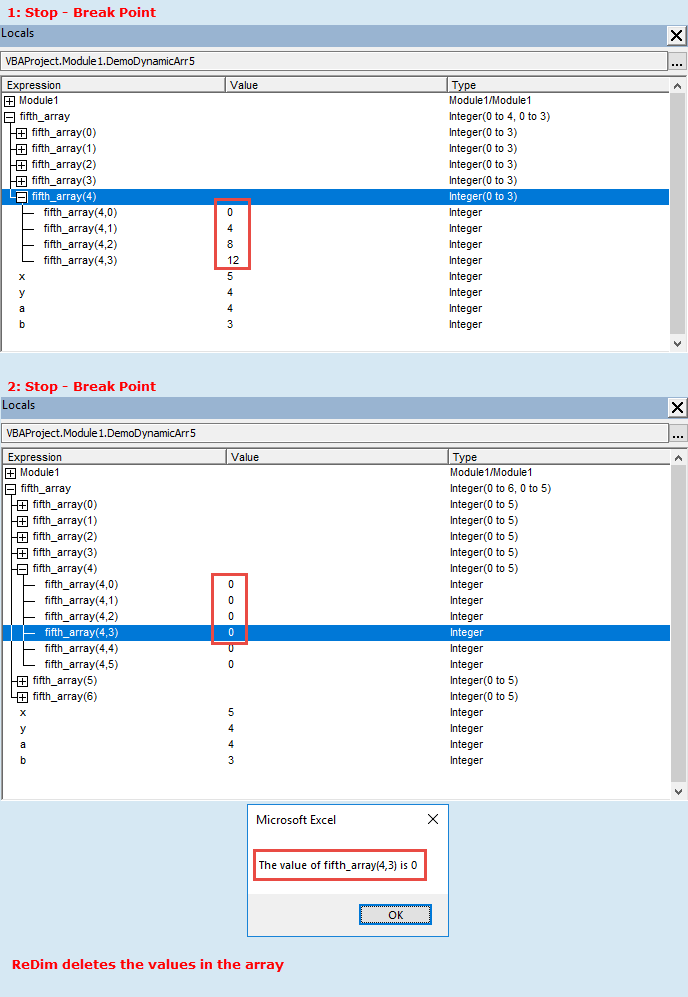Fig 7: Locals Window and Excel MsgBox fifth_array dimension values at 1: Stop Break Point showing assigned value, and 2: Stop Break Point where the ReDim statement has erased the contents. Dimensions 1 and 2 are resized in this example


Sub DemoDynamicArr6()
' Dynamic array :: a populated array is resized
'                  use the Preserve keyword to retain the values

Dim sixth_array() As Integer
Dim x As Integer, y As Integer
Dim a As Integer, b As Integer

a = 4
b = 3

ReDim sixth_array(1 To a, 1 To b) As Integer

For x = 1 To a
For y = 1 To b
sixth_array(x, y) = x * y
Next y
Next x

3:  Stop
ReDim Preserve sixth_array(1 To a, 1 To b + 2) As Integer

4:  Stop
MsgBox "The value of sixth_array(" & a & "," & b & ") is " & sixth_array(a, b)
End Sub

The array at two Break Points, and the output is shown in figure 8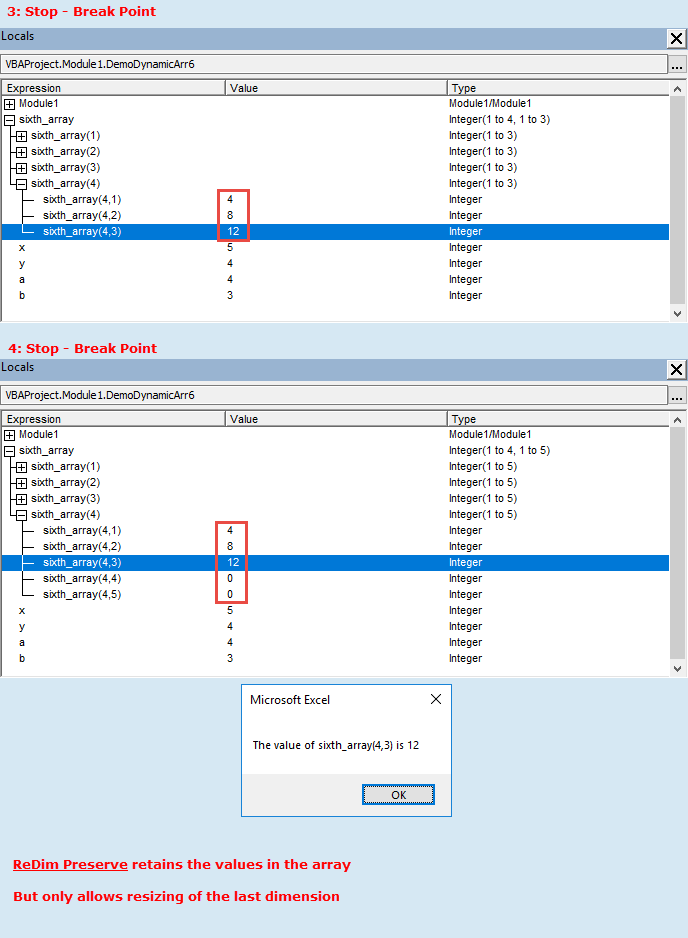Fig 8: Locals Window and Excel MsgBox six_array dimension values at 3: Stop Break Point showing assigned value, and 4: Stop Break Point where ReDim with the Preserve keyword has retained the contents. Only the last dimension, dimension 2 in this example is able to be resized


Sub DemoDynamicArr7()
' Dynamic array :: demonstrates the LBound and UBound functions

Dim seventh_array() As Integer
Dim x As Integer, y As Integer
Dim a As Integer, b As Integer
Dim LB1 As Long, UB1 As Long, LB2 As Long, UB2 As Long
Dim Prompt As String

a = 6: b = 2016

ReDim seventh_array(1 To a, 2012 To b) As Integer

For x = 1 To a
For y = 2012 To b
seventh_array(x, y) = x * y
Next y
Next x

ReDim Preserve seventh_array(1 To a, 2012 To b + 3)

LB1 = LBound(seventh_array): UB1 = UBound(seventh_array)
LB2 = LBound(seventh_array, 2): UB2 = UBound(seventh_array, 2)

Prompt = "Dimension 1 - lower bound:  " & LB1 & vbNewLine
Prompt = Prompt & "Dimension 1 - upper bound:  " & UB1 & vbNewLine
Prompt = Prompt & "Dimension 2 - lower bound:  " & LB2 & vbNewLine
Prompt = Prompt & "Dimension 2 - upper bound:  " & UB2

MsgBox Prompt, , "seventh_array :: dimension index numbers"
End Sub

' The array Type and MsgBox is shown in figure 9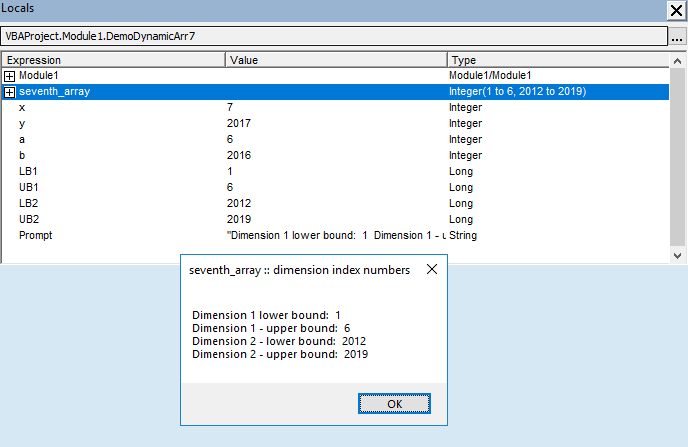Fig 9: Locals Window and Excel MsgBox dimension values after execution of the ReDim Preserve seventh_array(1 To a, 2012 To b + 3) statement

### Copy array to dynamic array withoutReDim

A dynamic array does not require a ReDim statement if you assign an initialized array, of the same data type, to it. See the examples in code 3.

Code 3: DemoDynamicArrAssignment
Sub DemoDynamicArrAssignment()
Dim ArrS1(1 To 5, 1 To 3) As String  ' static array
Dim ArrS2(1 To 3, 1 To 6) As String  ' static array
Dim ArrD() As String                 ' dynamic array
Dim i As Integer, j As Integer       ' loop counters

' write static array1
For i = 1 To UBound(ArrS1)
For j = 1 To UBound(ArrS1, 2)
ArrS1(i, j) = "(" & i & "," & j & ")"
Next j
Next i

' write static array2
For i = 1 To UBound(ArrS2)
For j = 1 To UBound(ArrS2, 2)
ArrS2(i, j) = "(" & i & "," & j & ")"
Next j
Next i

' assign array to undimensioned dynamic array
ArrD() = ArrS1()    ' 1. ArrD() is 5 x 3

' add 4 columns to the dynamic array
ReDim Preserve ArrD(LBound(ArrD) To UBound(ArrD), LBound(ArrD, 2) To UBound(ArrD, 2) + 4) ' 2. ArrD() is 5 x 7

' assign array to dimensioned dynamic array
ArrD() = ArrS2()    ' 3. ArrD() is 3 x 6
End Sub


1. Lines 161 and 162: Declare two static arrays, named ArrS1 and ArrS2, each of data type String
2. Lines 163: Declare a dynamic array named ArrD of data type String
3. Lines 169 and 176: Populate each element of the static array with its respective index coordinates in the form (1,1) (1,2),...(i,j)
4. Lines 181: Assign the ArrS1 static array with dimension 5 x 3 to the uninitialized dynamic array ArrD - figure 10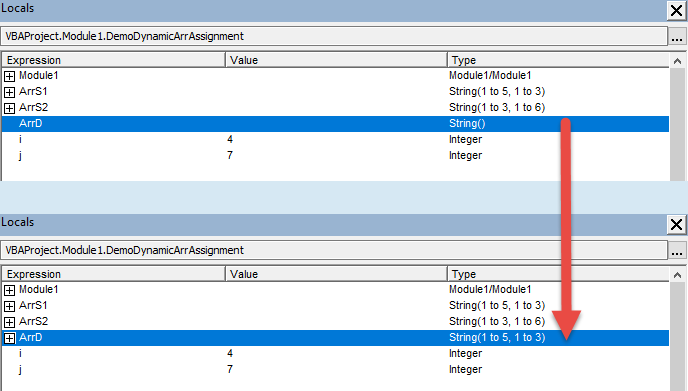Fig 10: Locals Window showing the ArrD array before and after execution of code line 181 ArrD() = ArrS1()
5. Lines 184: Redimension ArrD by adding 4 columns. The existing upper index number of the second dimension is returned by UBound(ArrD, 2). The result is shown in the upper panel of figure 11
6. Lines 187: Assign the ArrS2 static array with dimension 3 x 6 to the initialized dynamic array ArrD with existing dimension 5 x 7. ArrD is effectively re dimensioned to 3 x 6 - see figure 11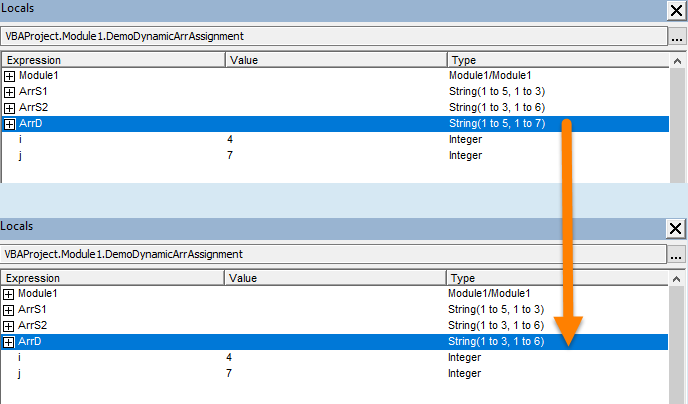Fig 11: Locals Window showing the ArrD array before and after execution of code line 187 ArrD() = ArrS2()

## References

Getz K, and M Gilbert, (2006), 'VBA Developer's Handbook'', Wiley

## Related material

Black Scholes on the HP10bII+ financial calculator

• Development platform: Excel 2016 (64 bit) Office 365 ProPlus and VBA 7.1
• Revised: Saturday 25th of February 2023 - 10:12 AM, [Australian Eastern Time (AET)]